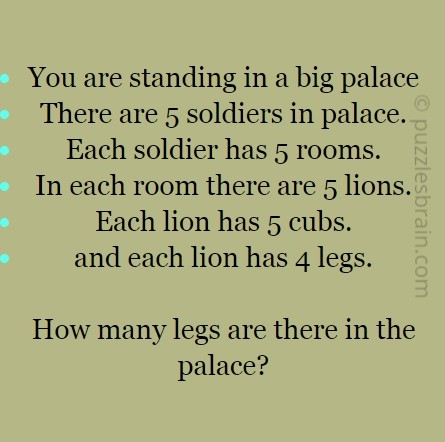Categories

# How Many Legs are in the Palace ?• You are standing in a big palace
• There are 5 soldiers in palace.
• Each soldier has 5 rooms.
• In each room there are 5 lions.
• Each lion has 5 cubs.
• and each lion has 4 legs.

How many legs are there in the palace?

Answer – How Many Legs are in the Palace ?

Explanation:

First, you have to get the total number of rooms within the palace. Since it says that there are 5 soldiers and each soldier has 5 rooms, so, there is a total of 25 rooms within the palace.

Number of Rooms = 5 x 5 = 25.

There are 5 lions in each room. If there are 5 lions in each room, and there are 25 rooms, multiply 5 by 25 and you will get 125 as the number of lions.

Number of lions = 25 x 5 = 125.

There are 5 cubs for each lion. 5 cubs x 125 lions, it is equal to 625.

Number of cubs= 125 x 5 = 625.

Now, since both lions and cubs have 4 legs each,

Total Number of lions= lions + cubs = 625 + 125 = 750.

So Number of legs for all the lions is

Lions legs = 750 x 4 = 3000.

Number of Legs of Soldiers = 5 x 2 = 10.

As you are standing in the palace we will have to count them too.

So to get total number of legs

Total Number of Legs = 3000 + 10 + 2

Total Number of Legs = 3012

Search Keywords:

Count legs in palace puzzle, legs in palace riddle, legs palace tigers puzzle, legs in palace counting puzzle answer